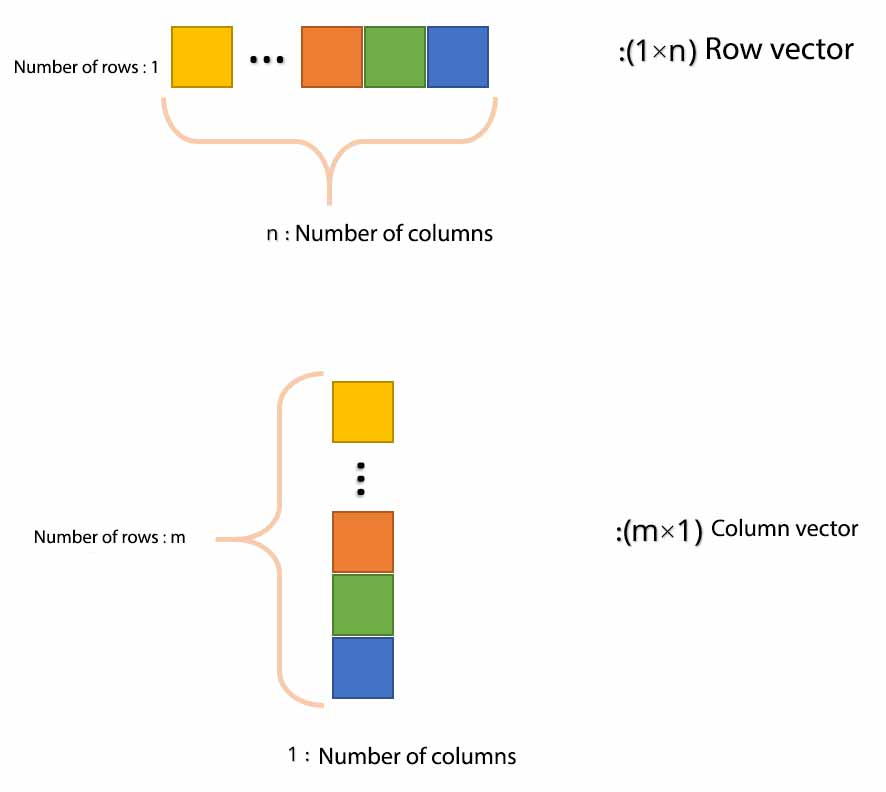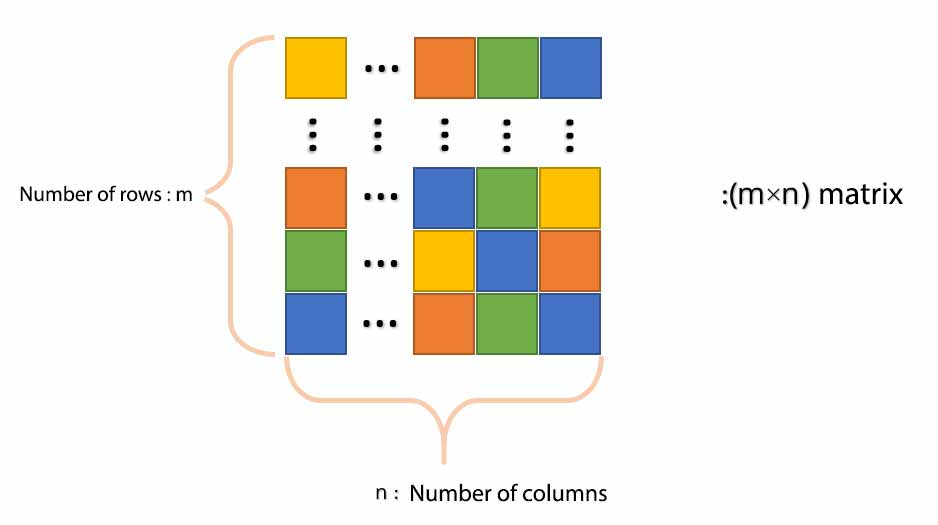MATLAB stands MATrix LABratory means Lab matrix well. The reason for this naming is that all MATLAB variables are arrays. That is, each variable can contain several elements. By array in MATLAB, we mean the same vector and matrix in MATLAB that you have definitely seen in mathematics. One of the most important parts of MATLAB is working with vectors and matrices in MATLAB. So familiarity with how to define and work with arrays in MATLAB is very important. The table of contents of this fourth session of free MATLAB training includes:

## Vector definition in MATLAB

One-dimensional arrays are called vectors. We have two types of vectors in MATLAB: row vector and column vector. A row vector has one row and several columns (image below, top row). In contrast, a column vector has multiple rows and a column (image below, bottom row). They use a m × n symbol to indicate the dimensions of the arrays. In this symbol, m represents the number of rows and n the number of columns. With this description, the line vector of an array with dimensions l 1 × n is the column vector of an array with dimensions m × 1. Next, in two separate sections, we define a line vector in MATLAB and a column vector in MATLAB.vectors matlab

### 1- Line vector in MATLAB

In MATLAB, you can create a vector using the bracket ([]). To define a vector in MATLAB, you can write vector elements in brackets. By elements, we mean the same numbers inside the vector. Then separate these elements with space (comma) or comma (,) . For example, we want to create a variable called x that is a row vector with elements 3 and 5. In this case, we must write:

```>> x = [3 5]
x =
3 5

```

MATLAB removed the bracket itself in the show, which does not matter. Now we separate the numbers inside the bracket with a comma:

```>> x = [3, 5]
x =
3 5

```

As you can see, the result is the same as before. That is, whether you use a space to define a vector or a comma, the result does not change. You are free to work with any sign you are comfortable with. Well, let’s go to the first exercise of the array session in MATLAB Do not forget, solve it yourself and then see the answer… Exercise 1: Create a line vector called x with two elements 7 and 9.

```>> x1 = [7, 9]

x1 =
7 9```

### 2- Column vector in MATLAB

What do we do to define a column vector? We also need to use brackets to write a column vector in MATLAB. But this time to separate elements instead of spaces or commas, we have to separate numbers with a semicolon (;). So let’s review once:

• We use brackets to define the vector in MATLAB.
• To define a line vector in MATLAB, we use a combination of brackets with spaces or commas.
• To define a column vector in MATLAB, we use a combination of a bracket with a semicolon.

So now let’s define a variable called y that contains a column vector with elements 1 and 3. To do this we must write:

```>> y = [1; 3]

y =     1     3

```

Another way to define a column matrix is ​​to use the Enter key. That means to press the Enter key to write the next column and then write the number. For example, for the definition of y mentioned above, we can write:

```>> y = [1
3]
y =
1
3

```

You saw that the result of the above two codes is the same. This writing style may not look interesting. Maybe it would be nicer if the numbers below were also written. To do this, after hitting Enter, hit space a few times to put the following numbers:

```>> y = [1  3]

y = 1 3```

Exercise 2: Define a column vector called a with two elements 7 and 9.
```>> a = [7; 9]
a =
7
9

```
Exercise 3: Create a line vector called b with values ​​of 3, 10 and 5.
```>> b = [3, 10, 5]
b =
3 10 5

```
Exercise 4: Make a column vector called c with values ​​of 8, 2 and 4.
```>> c = [8; 2; -4]
c =
8
2
-4

```

Note: Each element of a vector can be an algebraic operation or the output of a function. Provided that a result is a number. That is, for example, we can define a line vector whose first element is 6 * 3 and whose second element is pi / 2. To do this, just write:

```>> [6 * 3 pi / 2]
ans =

18.0000 1.5708

```

We mean that we must put a positive/negative number. By the way, according to the third free MATLAB training session, can you increase the number of high matrix display digits ?!

Exercise 5: Define a column with a name of your choice whose first element is pi ^ 2 (pi to power 2) and whose second element is the number neper. (Use the exp command to define the Napier number.)
```>> d = [pi ^ 2; exp (1)]
d =
9.8696
2.7183

```

## Matrix definition in MATLABIn the previous section, we learned how to define row and column vectors. In the last part of the array session in MATLAB, we want to learn the definition of a matrix together. Two semicolons and; They gave us the ability to create rows and columns. Now we can make a matrix from the combination of these. To create a matrix, we combine spaces and semicolons to create an array with several rows and columns. Two-dimensional arrays in a MATLAB are called matrices. Note that priority is given to rows, and for example, if we want to create a 3 × 2 matrix, we must define our matrix line by line. We have already learned that when we say that an array is m × n, that is, m has rows and n columns. That is, here we want to define a matrix that has 2 rows and 3 columns. Note how to define the following matrix:

```>> x = [3 4 5; 6 7 8]
x =
3 4 5
6 7 8```

Again, in the example above, we first defined the first line (ie, 5, 4, 3). Next with; Go to the next row and then enter the second row (ie 8 7 6). Now let’s define the above matrix in another way:

```>> x = [4 5 6
7 8 9]
x =
4 5 6
7 8 9
```

Defining a matrix in this way is probably much clearer to you. MATLAB is not particularly strict in defining matrices and vectors. So you can define an array with any of the methods mentioned that you are more comfortable with.

Note each element of the array in MATLAB (both vector and matrix), elements are known.

Note The objects of a matrix, like a vector, can be the result of an algebraic operation or the output of a function.

Exercise 6: Create a matrix called x_y with dimensions of 3 × 2 with arbitrary values.
```>> x_y = [7, 6, 5; 1, 9, 8]
x_y =
7 6 5
1 9 8```

Note There is flexibility when creating arrays in MATLAB. For example, all of the following methods are valid for creating an array:

```x = [7 9]
x = [7,9]
x = [7 , 9]
x = [7, 9]```

Note At the beginning of this session, we said that all variables in MATLAB are arrays. You may be wondering what the second session ( definition of a variable in MATLAB ) was? Those who were not arrays! The answer is, by the way, they are arrays too! But the arrays of a MATLAB element consider even the variable x = 5 as an array. But an array with an element or zero dimension is called this type of array in MATLAB Scalar

The following definitions to remember:

• The next zero array in MATLAB is called a scalar.

• A one-dimensional array in MATLAB is called a vector.

• The two-dimensional array in MATLAB is called a matrix.

• Do you think we have a 3D or 4D array? (Answer us in the comments)